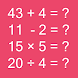# Math Game (Kids Math), MathematicsFree App

Rated 5.00/5 (8) —  Free Android application by Pregnancy

## About Math Game (Kids Math), Mathematics

Math game (Kids Math) is simple and small size android application designed with the aim to teach your kids, test your math skill and to workout with some set of arithmetic questions. You can call it a math workout game too.

Math game (Kids Math) is mainly for kids of age 4 to 12 year old.
Math game (Kids Math) is free game and best to test their math skill.
Learn with fun using Math game ! Mathematics game

Features

Math game addition test
Math game subtraction test
Math game multiplication test
Math game division test

In Math game there are twenty set of arithmetic addition test question. Questions are created instantly so there is no any chances of repetition of question. Some questions may be easy and some may be quite difficult .

Subtraction Test
In Math Game there are twenty set of arithmetic subtraction test question. Question are randomly created and there is no chances of repetition. Due to random generation some may be easy and some may be quite complex. You can teach your child very easily using this kids math game. Result of subtraction may be positive or negative.

Multiplication Test
In math game there is also multiplication test question. Where you can test your multiplication skill very easily. There are random set of multiplication number you have to select correct answer.

Division Test
Division test is also main part of this math game application. There are twenty set of division question.

Result of test is shown when you complete your twenty set of question and your score display at last.

Today every kids are familiar with latest smartphone so kids math skill can be grown using this app.

Workout your kids math skill using this free app.
Train your brain with math game ! Mathematics rocks !!!
For any feedback about Math game (Kids math ) mail at [email protected]

### Never Miss An Update!

Download and install Math Game (Kids Math), Mathematics version 1.1 on your Android device!
Android package: net.math.mathgame, download Math Game (Kids Math), Mathematics.apk

Free
downl.
Android
4.0.3+
For everyone
Android app

### App History & Updates

What's Changed
Math game Setting Update

## What are users saying about Math Game (Kids Math), Mathematics

Q70%
by Q####:

nice

B70%
by B####:

Application have very good mathematical problems which enbles children to acquire mathematical skills very easily. It is very good for children struggling for basic math.

M70%
by M####:

Great app. Now my son will increase his math skills. All thanks goes to developer .????????

P70%
by P####:

Bachhe ke liya aacha hai yai app, thank you developer my kids learning from your app.

X70%
by X####:

Sooo nice

O70%
by O####:

Mathematics app easy problems for child. hope it will helps in growing child education

K70%
by K####:

wow i used this app to teach my brother and he is much if interested now. He loves what he is doing as well as I too get a great time with this app. I recommend if you want to have math as you undetachable friend.

RATING
5.05
8 users

5

4

3

2

1